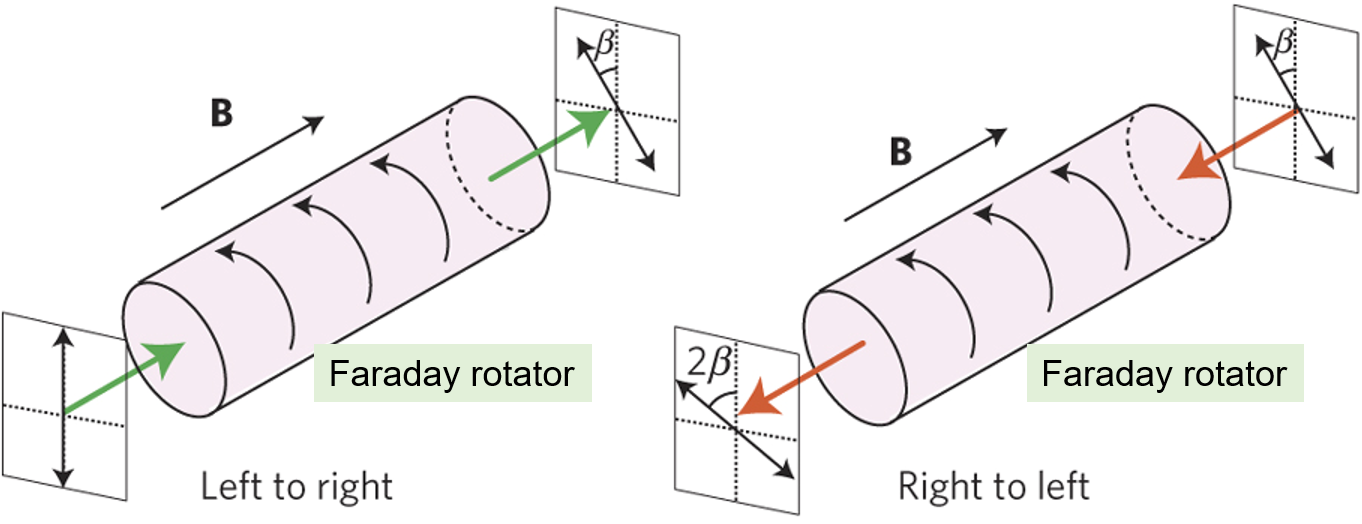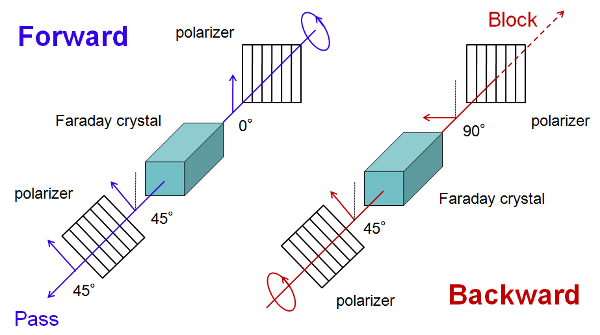## 波片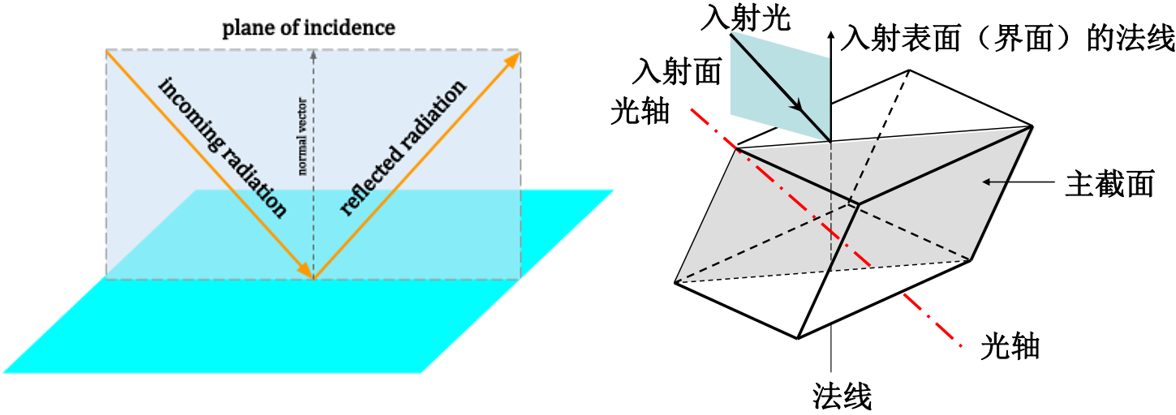Birefringence(双折射)的根源是晶体的各向异性，同时也体现了光的横波特性(纵波没有偏振)，晶体的光轴即光沿此方向入射时无双折射。(下图左侧图可能有误)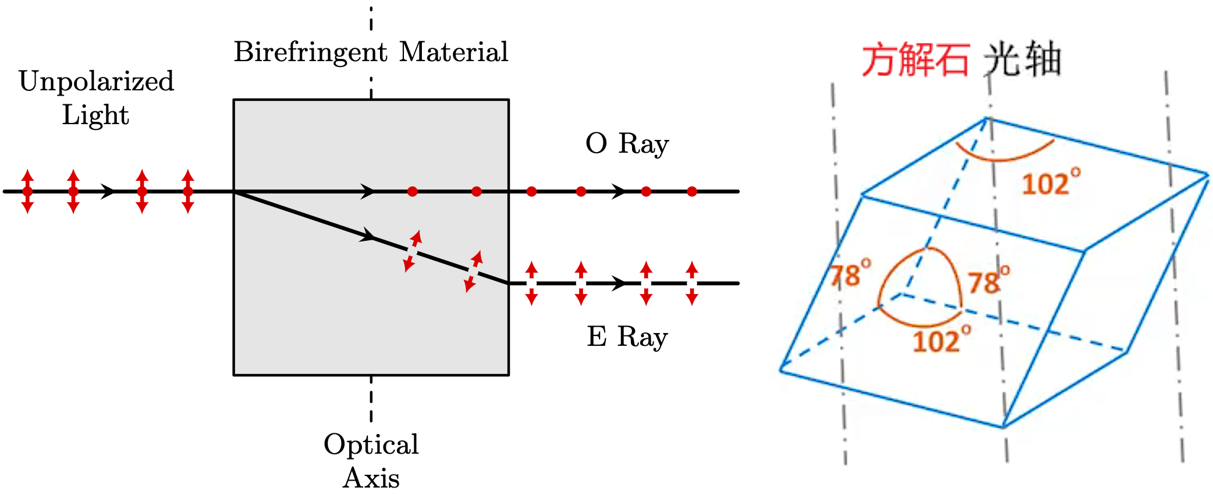• 所有方向都是光轴：在光学上是各向同性，没有双折射——立方晶体；
• 有双折射：
• 单轴晶体——三方、四方、六方晶体；
• 双轴晶体——三斜、单斜和正交晶系；

• 方解石、石英等，只有一个光轴；
• 在垂直于光轴的平面内是各向同性的(isotropic)；
• $$n_i \neq n_j=n_k$$，即光轴方向的折射率不同于其他两个结晶轴；
• 晶体一般是非磁性介质，因此在不同方向所展现的不同材料特性是介电常数，而不是磁导率；
• 快/慢轴：当光传播通过具有最小折射率的轴时，拥有更高的相速度，则该轴被称为快轴，反之，如果是通过具有最大折射率(最小相速度)的轴被称为慢轴
•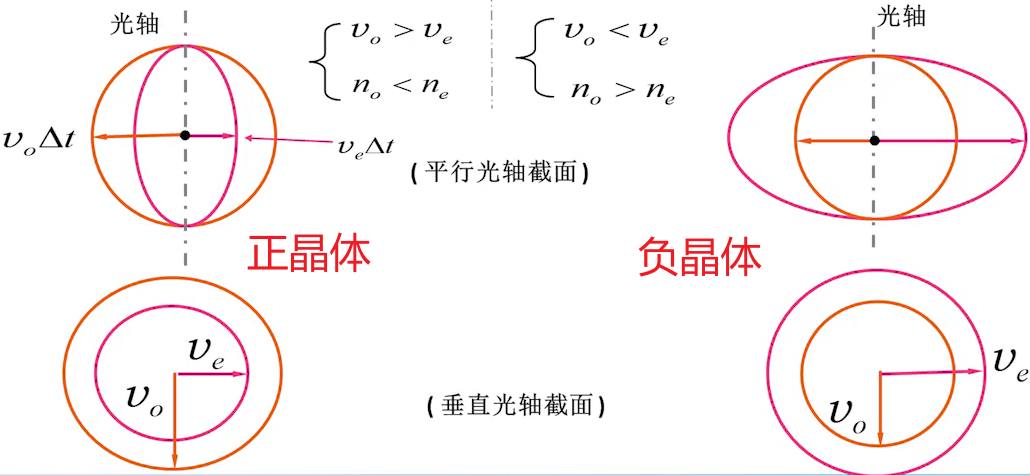• 正单轴晶体：比如石英SiO2，MgF2，金红石TiO2，$$n_e>n_o$$；
• 负单轴晶体：比如方解石CaCO3，红宝石Al2O3，$$n_e<n_o$$；
• 光沿着光轴方向传播 —— $$o$$光和$$e$$光感受到的折射率相同，传播速度相同；
• 光沿着垂直光轴的方向传播 —— $$o$$光和$$e$$光感受到的折射率不同，相速度不同，根据此原理可以制作波片

$$o$$光和$$e$$光：只针对单轴晶体或者双轴晶体而言(针对双折射现象而言)，出了晶体就不这样叫了。

• $$o$$光(寻常光，ordinary ray)，走正常折射路线的光，即完全符合折射定律，折射光和入射光在同一个平面内；
• $$e$$光(非常光，extraordinary ray)，走不正常折射路线的光，出现在双折射晶体中，入射角的正弦值与折射角的正弦值之比不是常数，而且折射光通常不在入射面内；
• $$o$$光主折射率为$$n_o=c/{v_o}$$，$$e$$光主折射率为$$n_e={v_e}$$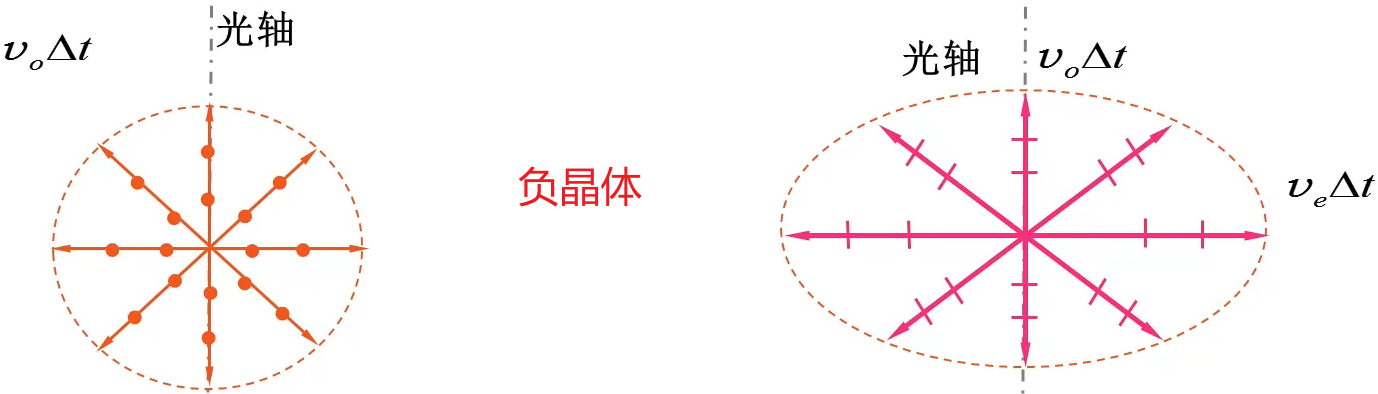• $$o$$光和$$e$$光都是线偏振光，而且偏振方向垂直，但是它们的传播方向是没有要求的。

• $$o$$光主平面：光轴与$$o$$光构成的的平面，$$o$$光的振动方向(电矢量)垂直于该平面；
• $$e$$光主平面：光轴与$$e$$光构成的的平面，$$e$$光的振动方向平行于该平面；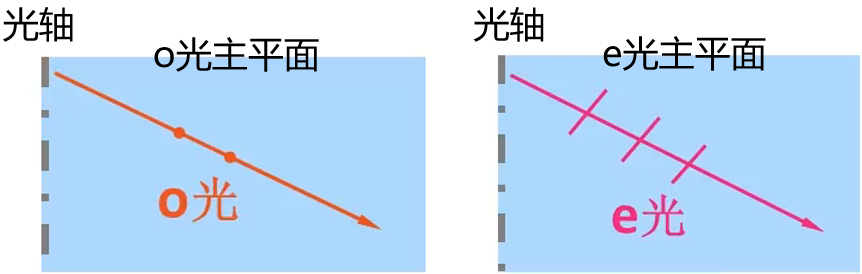• 波片制作是特定方向的(光轴平行于波片表面)，此时$$o$$光和$$e$$光方向不变，但是因为速度不同，产生相位差；
• 相位差的大小取决于波片的厚度，材料和工作波长。
• 晶体的光轴与入射表面平行；
• 平行偏振光正入射；
• 入射偏振和波片的快轴或慢轴平行，波片就不会改变偏振态，只是会产生对应的相位差；
• 波片的快轴：传播速度快的光矢量(light vector)的振动方向(轴)。负晶体的$$e$$轴(平行于光轴)，正晶体的$$o$$轴(垂直于光轴)；
• 波片的慢轴：传播速度慢的光的振动方向(轴)。负晶体的$$o$$轴，正晶体的$$e$$轴。
• 怎样区分波片的快慢轴？-Thorlabs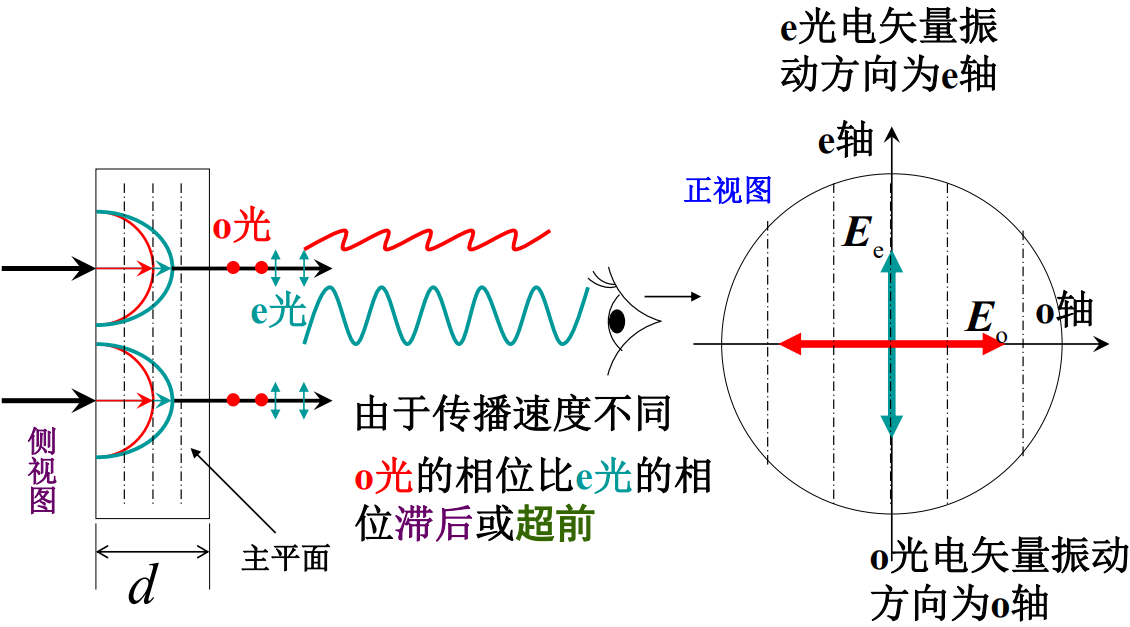• 波长问题——任何波片都是对特定波长而言的；
• 主轴方向问题——使用波片时应当知道波片允许的两个振动方向(即两个主轴方向)，及相应波速的快慢(哪个是快轴)，通常制作时已标定；
• 波片只改变偏振，不改变光强(忽略反射)——虽然波片对入射光的两个分量增加了相位差$$\delta$$，但在不考虑波片反射的情况下，因为振动方向垂直的光束不发生干涉，因此总光强与$$\delta$$无关，保持不变。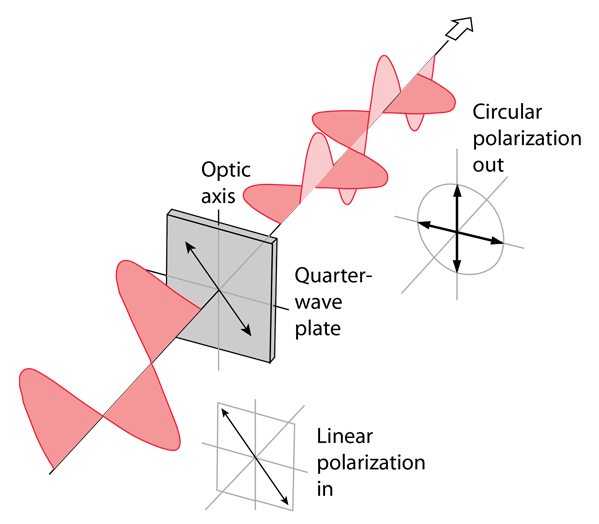四分之一波片(quarter-wave plate)：当光从法向入射透过波片时，寻常光($$o$$光)和非常光($$e$$光)之间的位相差等于$$\pi/2$$奇数倍，即产生对应的相位延迟。当线偏振光垂直入射$$1/4$$波片，根据入射线偏振光的偏振方向不同，出射后线偏振光变成椭圆偏振光、圆偏振光或者线偏振光；特别地当入射光的偏振和波片的快轴或慢轴成$$45^{\circ}$$角时，出射光为圆偏振光。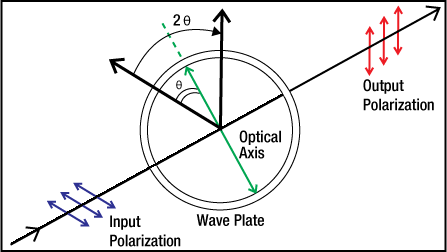二分之一波片(half-wave plate)：shifts the polarization direction of linearly polarized light. 该波片产生$$\pi$$奇数倍的相位延迟，线偏振光通过二分之一波片后仍是线偏振光；若入射线偏振光的振动方向与波片快轴（或慢轴）夹角为α，则出射线偏振光的振动方向向着快轴（或慢轴）的方向转过2α角度。圆偏振光入射时，出射光是旋向相反的圆偏振光。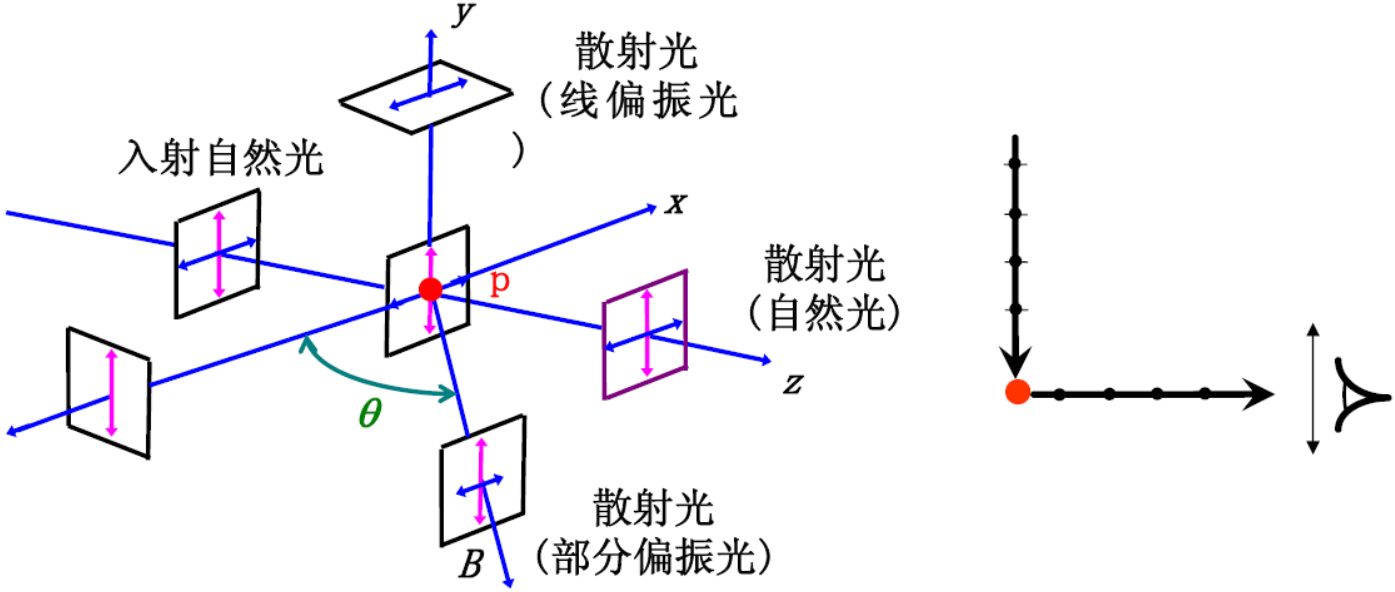偏振态 偏振片，透振方向绕光束旋转 先通过1/4波片，再通过偏振片 自然光 光强不变 光强不变(鉴定) 圆偏振光 光强不变 光强改变，出现消光(鉴定) 线偏振光 光强改变，在某一角度消光(鉴定) 部分偏振光 光强改变，但不消光 光强改变，但不消光 椭圆偏振光 光强改变，但不消光 转动1/4波片和偏振片，出现消光(鉴定)

(1)
(2) Introduction to WaveplatesQuarter-Wave Plate—Hyperphysics
(3) 晶体的双折射现象—B站
(4)
(5)

【S偏振】【P偏振】：S = Senkrecht，S偏振表示垂直偏振光，P = Parallel，P偏振表示平行偏振光。所谓的平行或者垂直是相对于偏振光学元件的入射平面而言的。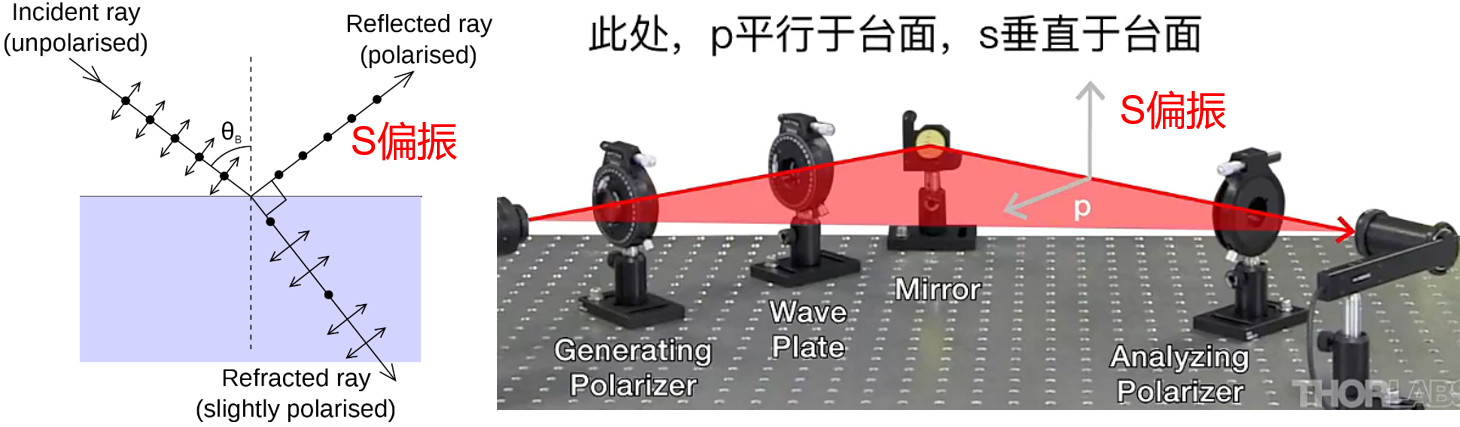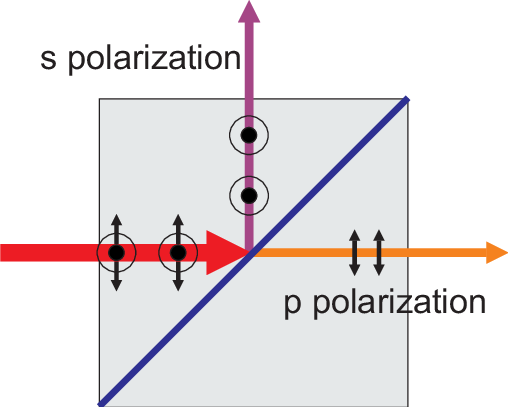TE模和TM模：TE模指的是横电波，TM模指的是横磁波 (都是针对光波导，在free space中我们一般不谈TE/TM/TEM的概念)

• TE: No electric field in the direction of propagation. These are sometimes called H modes because there is only a magnetic field along the direction of propagation (H is the conventional symbol for magnetic field).
• TM: No magnetic field in the direction of propagation. These are sometimes called E modes because there is only an electric field along the direction of propagation.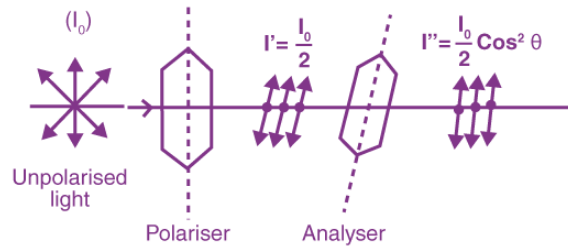马吕斯定律(Malus's Law)：偏振光照射于偏振片，二者之间的夹角为$$\theta$$，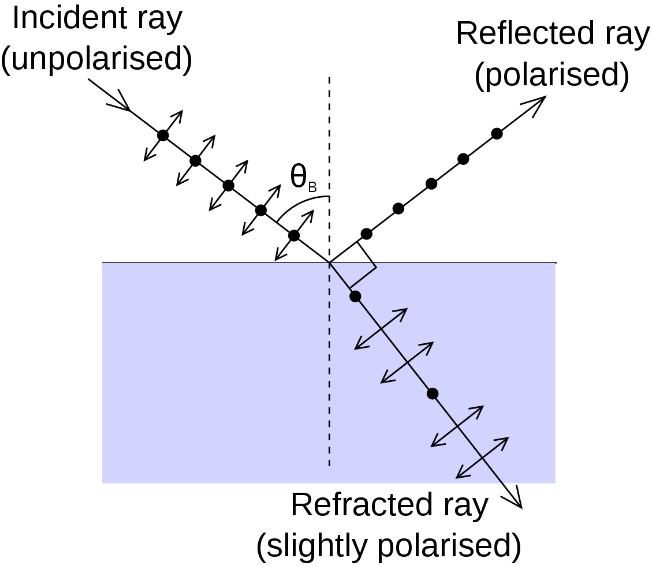布儒斯特角当入射自然光以此角度射入界面时，反射光是线偏振光，并且与折射光线互相垂直。$$n_1 \sin \theta_{\mathrm{B}}=n_2 \sin \left(90^{\circ}-\theta_{\mathrm{B}}\right)=n_2 \cos \theta_{\mathrm{B}}$$整理得到$$\theta_{B}=\arctan \left(\frac{n_{2}}{n_{1}}\right)$$

• 光的强度取决于坡印矢量($$E$$和$$B$$的叉乘)，而坡印矢量正比于$$E_{0}$$；
• 非偏振光，经过埃德温.兰德(Edwin Land)偏振片时，光子震动方向在偏振方向上投影，得到完全偏振光，对$$0$$到$$\pi / 2$$范围内取平均值，光强变为$$1/2$$($$\cos ^{2} \theta$$的积分)；
• 非偏振光经过偏振片后，得到的偏振光强为原来的$$1/2$$；再经过角度为$$\theta$$的偏振片后的光强为原来的$$\cos ^{2} \theta$$倍；
• 任何波长的偏振光，通过另一个旋转一定角度的偏振片后，强度发生变化，也就是能量发生了变化，我们知道能量和波长(或频率)相关，似乎意味着波长也发生了变化，但是实际上没有，蓝色偏振光入射，得到的依旧是蓝光。具体的需要量子力学的解释；
• 光的偏振方向一般用电场矢量去描述，当然你也可以选择磁场矢量，二者本质是一样的；
• 反射光里面偏振光的成分比较多(部分偏振)，特别是如果入射角恰好为布儒斯特角，此时反射光是完全偏振光，如果给相机安装偏振片，那么旋转到适当的角度，就可以尽量消除反射光的影响，从而拍清楚窗户或者水底的景象。
• s偏振和p偏振。

### 偏振的应用